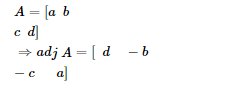# if the solve this problem`
Question:

If $S=\left[\begin{array}{ll}a & b \\ c & d\end{array}\right]$, then adj $A$ is

(a) $\left[\begin{array}{cc}-d & -b \\ -c & a\end{array}\right]$

(b) $\left[\begin{array}{cc}d & -b \\ -c & a\end{array}\right]$

(c) $\left[\begin{array}{ll}d & b \\ c & a\end{array}\right]$

(d) $\left[\begin{array}{ll}d & c \\ b & a\end{array}\right]$

Solution:

(b) $\left[\begin{array}{cc}d & -b \\ -c & a\end{array}\right]$

Adjoint of a square matrix of order 2 is obtained by interchanging the diagonal elements and changing the signs of off-diagonal elements.

Here,